339
Graph Theory - Part I
Graph
Graph Theory
CodeMonk

Introduction:
What is a graph? Do we use it a lot of times? Let’s think of an example: Facebook. The humongous network of you, your friends, family, their friends and their friends etc. are called as a social graph. In this "graph", every person is considered as a node of the graph and the edges are the links between two people. In Facebook, a friend of yours, is a bidirectional relationship, i.e., A is B's Friend => B is A's friend, so the graph is an Undirected Graph.

Nodes? Edges? Undirected? We’ll get to everything slowly.

Let’s redefine graph by saying that it is a collection of finite sets of vertices or nodes (V) and edges (E). Edges are represented as ordered pairs (u, v) where (u, v) indicates that there is an edge from vertex u to vertex v. Edges may contain cost, weight or length. The degree or valency of a vertex is the number of edges that connect to it. Graphs are of two types:
Undirected: Undirected graph is a graph in which all the edges are bidirectional, essentially the edges don’t point in a specific direction.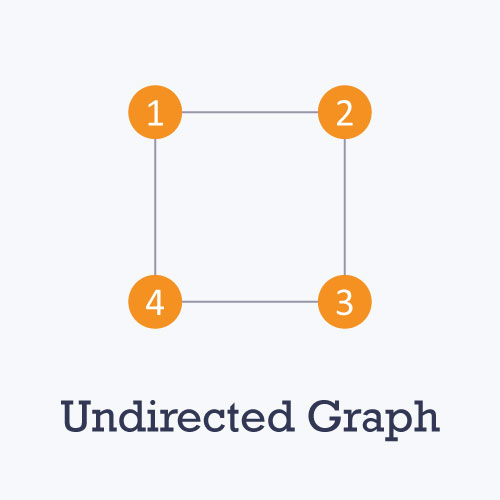Directed: Directed graph is a graph in which all the edges are unidirectional.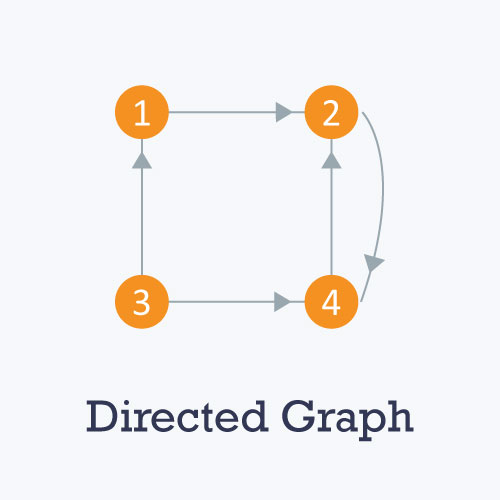A weighted graph is the one in which each edge is assigned a weight or cost. Consider a graph of 4 nodes as shown in the diagram below. As you can see each edge has a weight/cost assigned to it. Suppose we need to go from vertex 1 to vertex 3. There are 3 paths.
1 -> 2 -> 3
1 -> 3
1 -> 4 -> 3

The total cost of 1 -> 2 -> 3 will be (1 + 2) = 3 units.
The total cost of 1 -> 3 will be 1 units.
The total cost of 1 -> 4 -> 3 will be (3 + 2) = 5 units.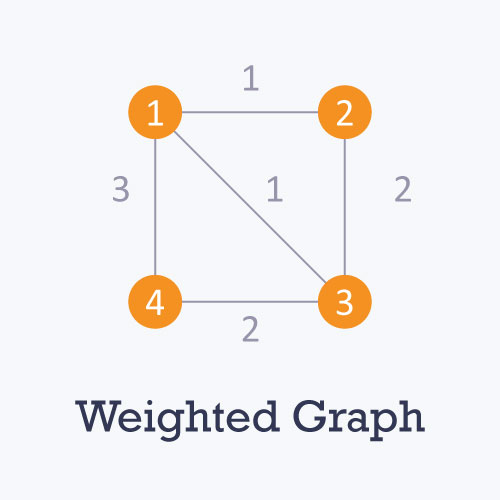A graph is called cyclic if there is a path in the graph which starts from a vertex and ends at the same vertex. That path is called a cycle. An acyclic graph is a graph which has no cycle.

A tree is an undirected graph in which any two vertices are connected by only one path. Tree is acyclic graph and has N - 1 edges where N is the number of vertices.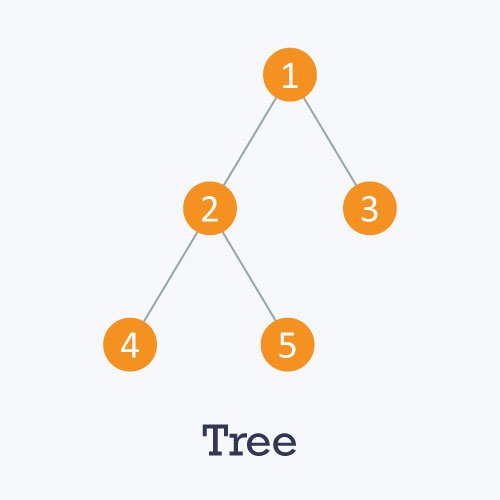Graph Representation:

There are variety of ways to represent a graph. Two of them are:

Adjacency Matrix: An adjacency matrix is a V x V binary matrix A (a binary matrix is a matrix in which the cells can have only one of two possible values - either a 0 or 1). Element Ai,j is 1 if there is an edge from vertex i to vertex j else Ai,j is 0. The adjacency matrix can also be modified for the weighted graph in which instead of storing 0 or 1 in Ai,j we will store the weight or cost of the edge from vertex i to vertex j. In an undirected graph, if Ai,j = 1 then Aj,i = 1. In a directed graph, if Ai,j = 1 then Aj,i may or may not be 1. Adjacency matrix providers constant time access (O(1) ) to tell if there is an edge between two nodes. Space complexity of adjacency matrix is O(V2).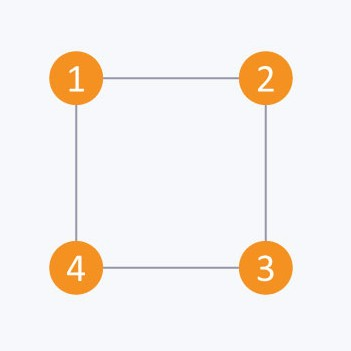The adjacency matrix of above graph is:
i/j : 1 2 3 4
1 : 0 1 0 1
2 : 1 0 1 0
3 : 0 1 0 1
4 : 1 0 1 0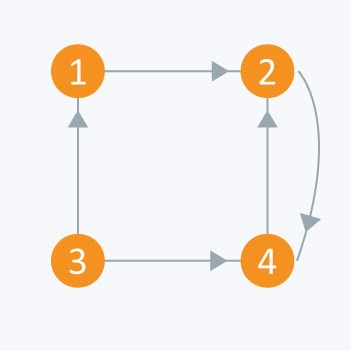The adjacency matrix of above graph is:

i/j: 1 2 3 4
1 : 0 1 0 0
2 : 0 0 0 1
3 : 1 0 0 1
4 : 0 1 0 0

Consider the above directed graph and let us create this graph using an Adjacency matrix and then show all the edges that exist in the graph.

Input File:
4
5
1 2
2 4
3 1
3 4
4 2

Code:

#include <iostream>

using namespace std;

bool A;

void initialize()
{
for(int i = 0;i < 10;++i)
for(int j = 0;j < 10;++j)
A[i][j] = false;
}

int main()
{
int x, y, nodes, edges;
initialize();       // Since there is no edge initially
cin >> nodes;       // Number of nodes
cin >> edges;       // Number of edges
for(int i = 0;i < edges;++i)
{
cin >> x >> y;
A[x][y] = true;     // mark the edges from vertex x to vertex y
}
if(A == true)
cout << “There is an edge between 3 and 4” << endl;
else
cout << “There is no edge between 3 and 4” << endl;

if(A == true)
cout << “There is an edge between 2 and 3” << endl;
else
cout << “There is no edge between 2 and 3” << endl;

return 0;
}


Output:
There is an edge between 3 and 4
There is no edge between 2 and 3

Adjacency List: The other way to represent a graph is an adjacency list. Adjacency list is an array A of separate lists. Each element of the array Ai is a list which contains all the vertices that are adjacent to vertex i. For weighted graph we can store weight or cost of the edge along with the vertex in the list using pairs. In an undirected graph, if vertex j is in list Ai then vertex i will be in list Aj. Space complexity of adjacency list is O(V + E) because in Adjacency list we store information for only those edges that actually exist in the graph. In a lot of cases, where a matrix is sparse (A sparse matrix is a matrix in which most of the elements are zero. By contrast, if most of the elements are nonzero, then the matrix is considered dense.) using an adjacency matrix might not be very useful, since it’ll use a lot of space where most of the elements will be 0, anyway. In such cases, using an adjacency list is better.Consider the same undirected graph from adjacency matrix. Adjacency list of the graph is:

A1 → 2 → 4
A2 → 1 → 3
A3 → 2 → 4
A4 → 1 → 3Consider the same graph from adjacency matrix. Adjacency list of the graph is:

A1 → 2
A2 → 4
A3 → 1 → 4
A4 → 2

Consider the above directed graph and let’s code it.

Input File:
4
5
1 2
2 4
3 1
3 4
4 2

Code:

 #include<iostream >
#include < vector >

using namespace std;

int main()
{
int x, y, nodes, edges;
cin >> nodes;       // Number of nodes
cin >> edges;       // Number of edges
for(int i = 0;i < edges;++i)
{
cin >> x >> y;
}
for(int i = 1;i <= nodes;++i)
{
cout << "Adjacency list of node " << i << ": ";
for(int j = 0;j < adj[i].size();++j)
{
else
cout << adj[i][j] << " --> ";
}
}
return 0;
}


Output:
Adjacency list of node 1: 2
Adjacency list of node 2: 4
Adjacency list of node 3: 1 --> 4
Adjacency list of node 4: 2

Graph Traversals:

While using some graph algorithms, we need that every vertex of a graph should be visited exactly once. The order in which the vertices are visited may be important, and may depend upon the particular algorithm or particular question which we’re trying to solve. During a traversal, we must keep track of which vertices have been visited. The most common way is to mark the vertices which have been visited.

So, graph traversal means visiting every vertex and every edge exactly once in some well-defined order. There are many approaches to traverse the graph. Two of them are:

Depth First Search (DFS):

Depth first search is a recursive algorithm that uses the idea of backtracking. Basically, it involves exhaustive searching of all the nodes by going ahead - if it is possible, otherwise it will backtrack. By backtrack, here we mean that when we do not get any further node in the current path then we move back to the node,from where we can find the further nodes to traverse. In other words, we will continue visiting nodes as soon as we find an unvisited node on the current path and when current path is completely traversed we will select the next path.

This recursive nature of DFS can be implemented using stacks. The basic idea is that we pick a starting node and push all its adjacent nodes into a stack. Then, we pop a node from stack to select the next node to visit and push all its adjacent nodes into a stack. We keep on repeating this process until the stack is empty. But, we do not visit a node more than once, otherwise we might end up in an infinite loop. To avoid this infinite loop, we will mark the nodes as soon as we visit it.

Pseudocode :

DFS-iterative (G, s):                                          //where G is graph and s is source vertex.
let S be stack
S.push( s )            // inserting s in stack
mark s as visited.
while ( S is not empty):
// pop a vertex from stack to visit next
v  =  S.top( )
S.pop( )
//push all the neighbours of v in stack that are not visited
for all neighbours w of v in Graph G:
if w is not visited :
S.push( w )
mark w as visited

DFS-recursive(G, s):
mark s as visited
for all neighbours w of s in Graph G:
if w is not visited:
DFS-recursive(G, w)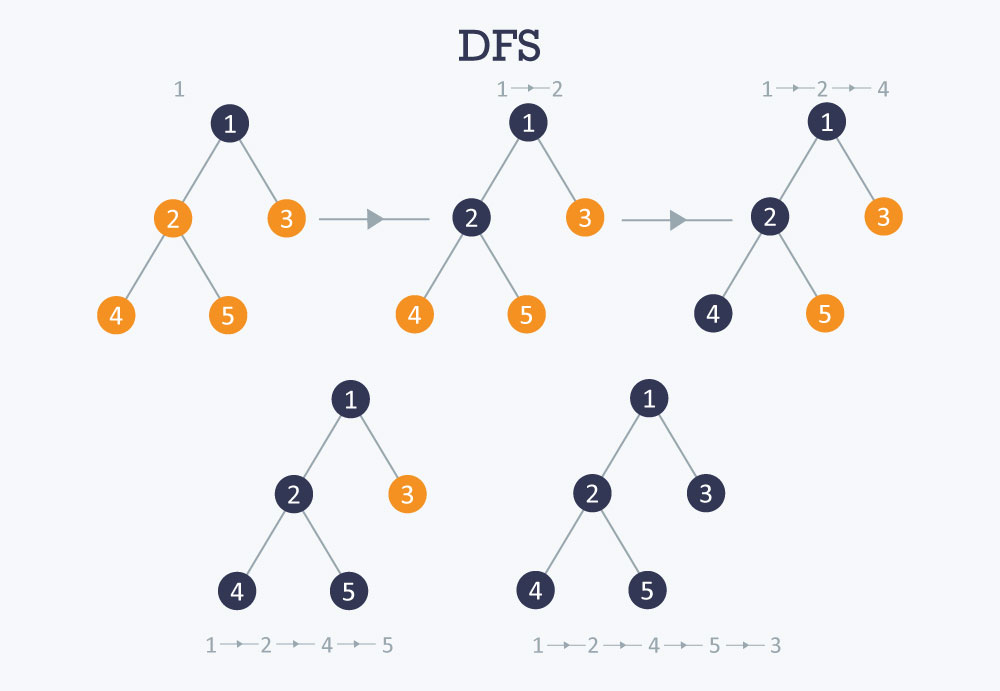Applications:

1) How to find connected components using DFS?

A graph is said to be disconnected if it is not connected, i.e., if there exist two nodes in the graph such that there is no edge between those nodes. In an undirected graph, a connected component is a set of vertices in a graph that are linked to each other by paths. Consider an example given in the diagram. As we can see graph G is a disconnected graph and has 3 connected components. First connected component is 1 -> 2 -> 3 as they are linked to each other. Second connected component 4 -> 5 and third connected component is vertex 6. In DFS, if we start from a start node it will mark all the nodes connected to start node as visited. So if we choose any node in a connected component and run DFS on that node it will mark the whole connected component as visited. So we will repeat this process for other connected components.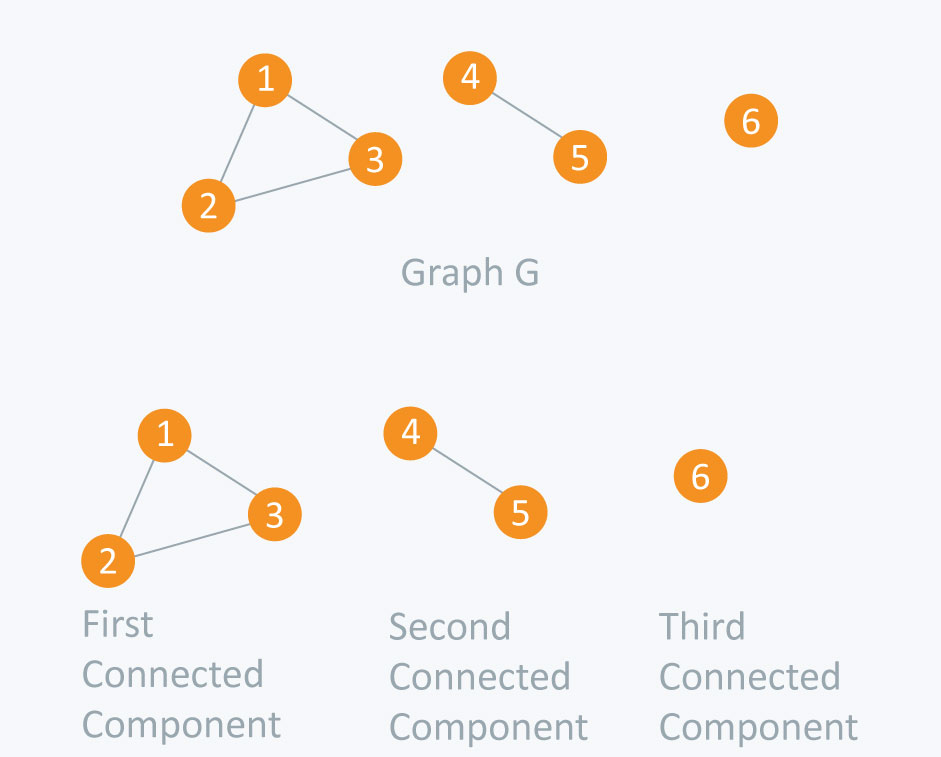Input File:
6
4
1 2
2 3
1 3
4 5

Code:

 #include <iostream>
#include <vector>
using namespace std;

bool visited;

void dfs(int s) {
visited[s] = true;
for(int i = 0;i < adj[s].size();++i)    {
}
}

void initialize() {
for(int i = 0;i < 10;++i)
visited[i] = false;
}

int main() {
int nodes, edges, x, y, connectedComponents = 0;
cin >> nodes;                       // Number of nodes
cin >> edges;                       // Number of edges
for(int i = 0;i < edges;++i) {
cin >> x >> y;
// Undirected Graph
adj[x].push_back(y);                   // Edge from vertex x to vertex y
adj[y].push_back(x);                   // Edge from vertex y to vertex x
}

initialize();                           // Initialize all nodes as not visited

for(int i = 1;i <= nodes;++i) {
if(visited[i] == false)     {
dfs(i);
connectedComponents++;
}
}
cout << "Number of connected components: " << connectedComponents << endl;
return 0;
}


Output:
Number of connected components: 3

Its a traversing algorithm, where we start traversing from selected node (source or starting node) and traverse the graph layerwise which means it explores the neighbour nodes (nodes which are directly connected to source node) and then move towards the next level neighbour nodes. As the name suggests, we move in breadth of the graph, i.e., we move horizontally first and visit all the nodes of the current layer and then we move to the next layer.
Consider the diagram below: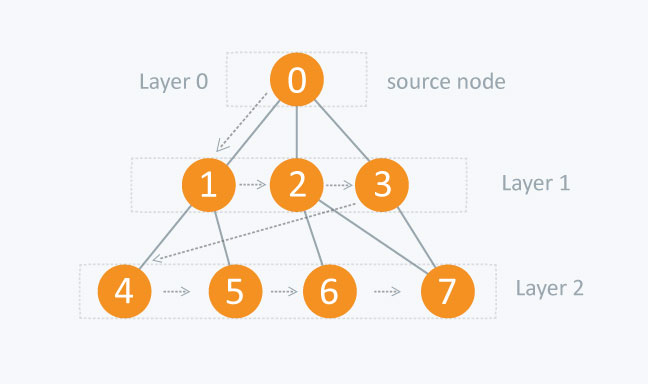In BFS, all nodes on layer 1 will be traversed before we move to nodes of layer 2. As the nodes on layer 1 have less distance from source node when compared with nodes on layer 2.
As the graph can contain cycles, so we may come at same node again while traversing the graph. So to avoid processing of same node again, we use a boolean array which marks the node marked if we have process that node. While visiting all the nodes of current layer of graph, we will store them in such a way, so that we can visit the children of these nodes in the same order as they were visited.

In the above diagram, starting from 0, we will visit its children 1, 2, and 3 and store them in the order they get visited. So that after visiting all the vertices of current layer, we can visit the children of 1 first(that are 4 and 5), then of 2 (that are 6 and 7) and then of 3(that is 7) and so on.

To make the above process easy, we will use a queue to store the node and mark it as visited, and we will store it in queue until all its neighbours (vertices which are directly connected to it) are marked. As queue follow FIFO order (First In First Out), it will first visit the neighbours of that node, which were inserted first in the queue.

Pseudocode :

BFS (G, s)                   //where G is graph and s is source node.
let Q be queue.
Q.enqueue( s ) // inserting s in queue until all its neighbour vertices are marked.

mark s as visited.
while ( Q is not empty)
// removing that vertex from queue,whose neighbour will be visited now.
v  =  Q.dequeue( )

//processing all the neighbours of v
for all neighbours w of v in Graph G
if w is not visited
Q.enqueue( w )             //stores w in Q to further visit its neighbour
mark w as visited.


For the Graph below: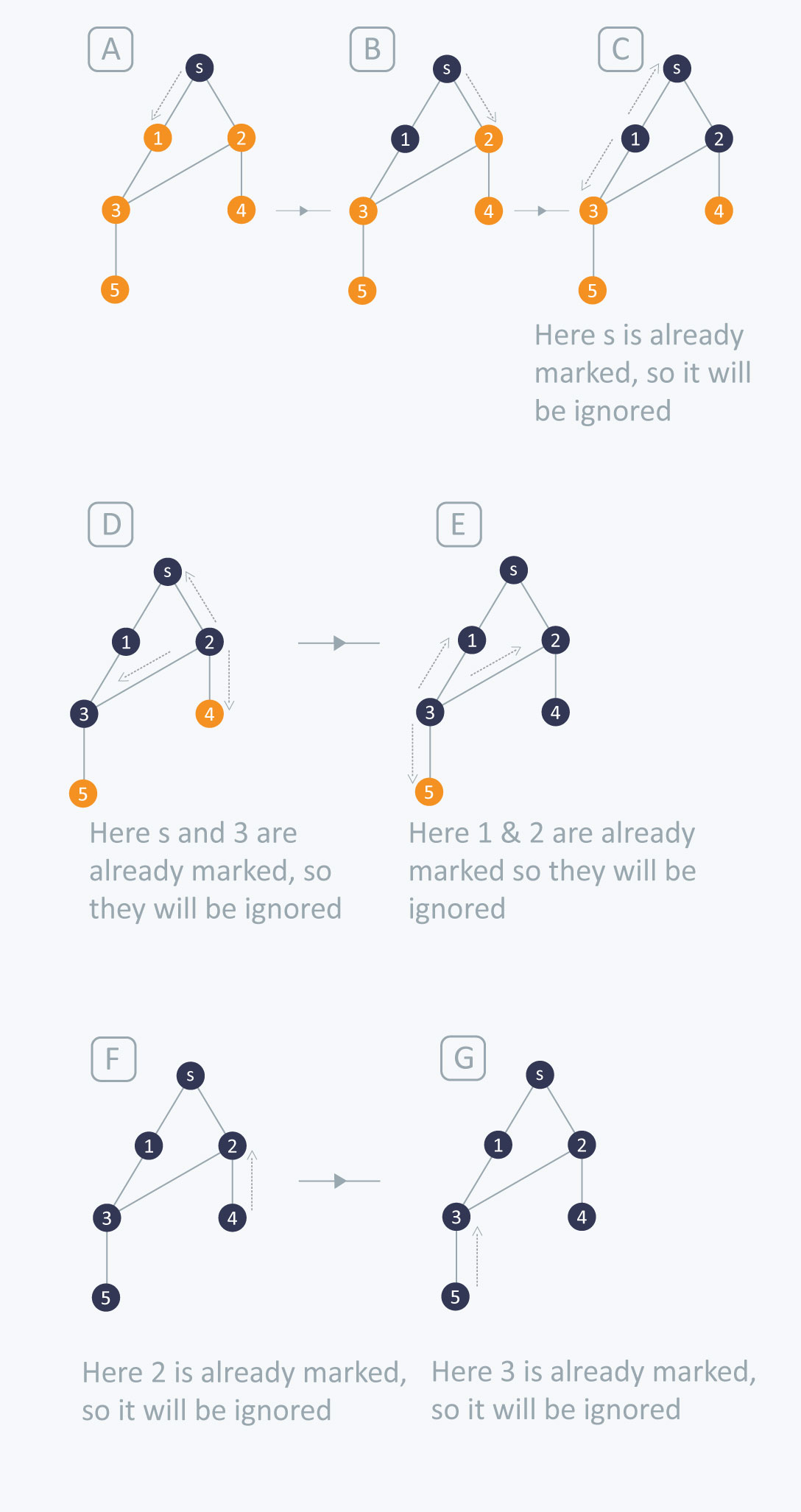Initially, it will start from the source node and will push s in queue and mark s as visited.

In first iteration, It will pop s from queue and then will traverse on neighbours of s that are 1 and 2. As 1 and 2 are unvisited, they will be pushed in queue and will be marked as visited.

In second iteration, it will pop 1 from queue and then will traverse on its neighbours that are s and 3. As s is already marked so it will be ignored and 3 is pushed in queue and marked as visited.

In third iteration, it will pop 2 from queue and then will traverse on its neighbours that are s,3 and 4. As 3 and s are already marked so they will be ignored and 4 is pushed in queue and marked as visited.

In fourth iteration, it will pop 3 from queue and then will traverse on its neighbours that are 1, 2 and 5. As 1 and 2 are already marked so they will be ignored and 5 is pushed in queue and marked as visited.

In fifth iteration, it will pop 4 from queue and then will traverse on its neighbours that is 2 only. As 2 is already marked so it will be ignored.

In sixth iteration, it will pop 5 from queue and then will traverse on its neighbours that is 3 only. As 3 is already marked so it will be ignored.

Now the queue is empty so it comes out of the loop.

Through this you can traverse all the nodes using BFS.

BFS can be used in finding minimum distance from one node of graph to another, provided all the edges in graph have same weight.
Example: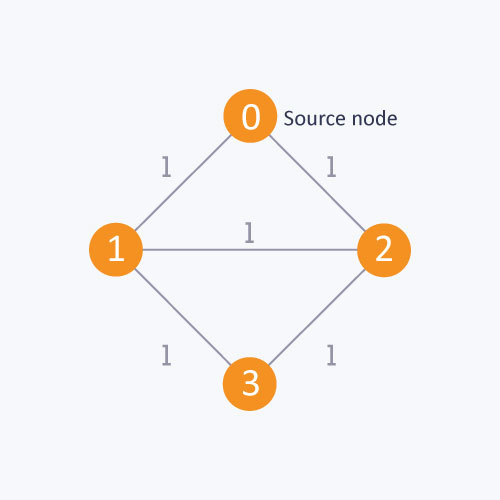As in the above diagram, starting from source node, to find the distance between 0 and 1, if we do not follow BFS algorithm, we can go from 0 to 2 and then to 1. It will give the distance between 0 and 1 as 2. But the minimum distance is 1 which can be obtained by using BFS.

Complexity: Time complexity of BFS is O(V + E) , where V is the number of nodes and E is the number of Edges.

Applications:

1) How to determine the level of each node in the given tree ?

As we know in BFS, we traverse level wise, i.e first we visit all the nodes of one level and then visit to nodes of another level. We can use BFS to determine level of each node.

Implementation:

vector <int> v ;   // vector for maintaining adjacency list explained above.
int level; // to determine the level of each node
bool vis; //mark the node if visited
void bfs(int s) {
queue <int> q;
q.push(s);
level[ s ] = 0 ;  //setting the level of sources node as 0.
vis[ s ] = true;
while(!q.empty())
{
int p = q.front();
q.pop();
for(int i = 0;i < v[ p ].size() ; i++)
{
if(vis[ v[ p ][ i ] ] == false)
{
//setting the level of each node with an increment in the level of parent node
level[ v[ p ][ i ] ] = level[ p ]+1;
q.push(v[ p ][ i ]);
vis[ v[ p ][ i ] ] = true;
}
}
}
}


Above code is similar to bfs except one change and i.e
level[ v[ p ][ i ] ] = level[ p ]+1;

here, when visiting each node, we set the level of that node with an increment in the level of its parent node .

Through this we can determine the level of each node.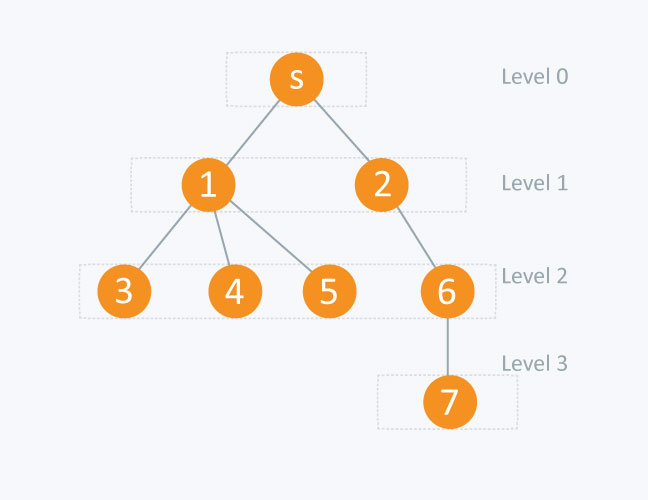In the diagram above :

          node      level[ node ]

s(source node)                      0
1                            1
2                            1
3                            2
4                            2
5                            2
6                            2
7                            3


2) 0-1 BFS: This type of BFS is used when we have to find the shortest distance from one node to another in a graph provided the edges in graph have weights 0 or 1. As if we apply the normal BFS explained above, it can give wrong results for optimal distance between 2 nodes.(explained below)
In this approach we will not use boolean array to mark the node visited as while visiting each node we will check the condition of optimal distance.
We will use Double Ended Queue to store the node.
In 0-1 BFS, if the edge is encountered having weight = 0, then the node is pushed to front of dequeue and if the edge’s weight =1, then it will be pushed to back of dequeue.

Implementation:
Here :

edges[ v ] [ i ] is an adjacency list that will exists in pair form i.e edges[ v ][ i ].first will contains the number of node to which v is connected and edges[ v ][ i ].second will contain the distance between v and edges[ v ][ i ].first .
Q is double ended queue.
distance is an array where, distance [ v ] will contain the distance from start node to v node.
Initially define distance from source node to each node as infinity.

 void bfs (int start)
{
deque <int > Q;     // double ended queue
Q.push_back( start);
distance[ start ] = 0;
while( !Q.empty ())
{
int v = Q.front( );
Q.pop_front();
for( int i = 0 ; i < edges[v].size(); i++)
{
/* if distance of neighbour of v from start node is greater than sum of distance of v from start node and edge weight between v and its neighbour (distance between v and its neighbour of v) ,then change it */
if(distance[ edges[ v ][ i ].first ] > distance[ v ] + edges[ v ][ i ].second )
{

distance[ edges[ v ][ i ].first ] = distance[ v ] + edges[ v ][ i ].second;
/*if edge weight between v and its neighbour is 0 then push it to front of
double ended queue else push it to back*/
if(edges[ v ][ i ].second == 0)
{
Q.push_front( edges[ v ][ i ].first);
}
else
{
Q.push_back( edges[ v ][ i ].first);

}
}
}


Let’s understand the above code with the Graph given below: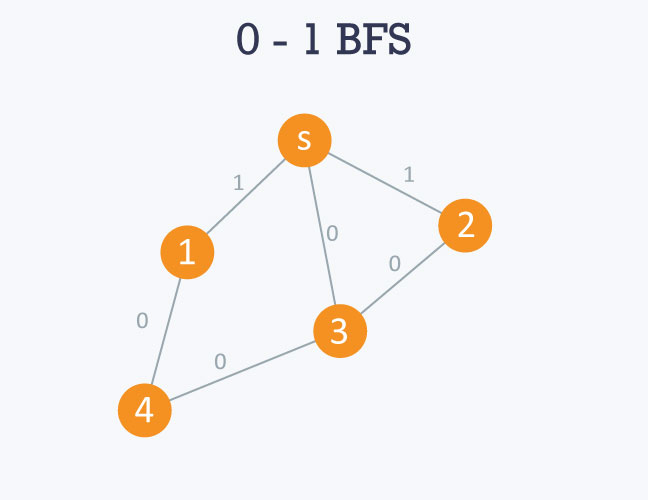Adjacency List of above graph will be:
here s can be taken as 0 .
0 -> 1 -> 3 -> 2
edges[ 0 ][ 0 ].first = 1 , edges[ 0 ][ 0 ].second = 1
edges[ 0 ][ 1 ].first = 3 , edges[ 0 ][ 1 ].second = 0
edges[ 0 ][ 2 ].first = 2 , edges[ 0 ][ 2 ].second = 1

1 -> 0 -> 4
edges[ 1 ][ 0 ].first = 0 , edges[ 1 ][ 0 ].second = 1
edges[ 1 ][ 1 ].first = 4 , edges[ 1 ][ 1 ].second = 0

2 -> 0 -> 3
edges[ 2 ][ 0 ].first = 0 , edges[ 2 ][ 0 ].second = 0
edges[ 2 ][ 1 ].first = 3 , edges[ 2 ][ 1 ].second = 0

3 -> 0 -> 2 -> 4
edges[ 3 ][ 0 ].first = 0 , edges[ 3 ][ 0 ].second = 0
edges[ 3 ][ 2 ].first = 2 , edges[ 3 ][ 2 ].second = 0
edges[ 3 ][ 3 ].first = 4 , edges[ 3 ][ 3 ].second = 0

4 -> 1 -> 3
edges[ 4 ][ 0 ].first = 1 , edges[ 4 ][ 0 ].second = 0
edges[ 4 ][ 1 ].first = 3 , edges[ 4 ][ 1 ].second = 0

So if we use normal bfs here, it will give us wrong results by showing optimal distance between s and 1 node as 1 and between a and 2 as 1, but the real optimal distance is 0 in both the cases. As it simply visits the children of s and calculate the distance between s and its children which will give 1 as the answer.

Processing:

Initiating from source node, i.e 0, it will move towards 1,2 and 3. As the edge weight between 0 and 1 is 1 and between 0 and 2 is 1 , so they will be pushed in back side of queue and as edge weight between 0 and 3 is 0, so it will pushed in front side of queue. Accordingly, distance will be maintained in distance array.
Then 3 will be popped up from queue and same process will be applied on its neighbours and so on..

3) Peer to Peer Networks: In peer to peer networks, Breadth First Search is used to find all neighbour nodes.
4) GPS Navigation systems: Breadth First Search is used to find all neighboring locations.
5) Broadcasting in Network: In networks, a broadcasted packet follows Breadth First Search to reach all nodes.